# Computational homogenization of transient chemo-mechanical processes based on a variational minimization principle

## Abstract

We present a variational framework for the computational homogenization of chemo-mechanical processes of soft porous materials. The multiscale variational framework is based on a minimization principle with deformation map and solvent flux acting as independent variables. At the microscopic scale we assume the existence of periodic representative volume elements (RVEs) that are linked to the macroscopic scale via first-order scale transition. In this context, the macroscopic problem is considered to be homogeneous in nature and is thus solved at a single macroscopic material point. The microscopic problem is however assumed to be heterogeneous in nature and thus calls for spatial discretization of the underlying RVE. Here, we employ Raviart–Thomas finite elements and thus arrive at a conforming finite-element formulation of the problem. We present a sequence of numerical examples to demonstrate the capabilities of the multiscale formulation and to discuss a number of fundamental effects.

## Introduction

The continuous advancement of technological innovations in the fields of digitalization and automation leads to increased demands of smart and multifunctional materials. In that context, the design of associated materials with tailor-made properties is paramount. Classical examples cover solids with coupled electro- and magneto-mechanical response such as ferroelectrics or magnetostrictives. More recently, several advanced materials exhibiting chemo-mechanical coupling have entered the scene and experience increased attention since then. Related materials show interactions between fluid flow and deformations and play an important role in emerging applications such as lithium-ion batteries (Wang et al. ), heterogeneous concretes (Wang and Ueda ), engineered biological tissues (Truskey et al. ), or fiber-reinforced superabsorbent hydrogels (Chen and Park ).

In order to allow for the theoretical development of related materials and structures, reliable continuum-mechanical models are needed. Related formulations have to take into account the mutual couplings between the fluidic diffusion of some solvent phase and the mechanical deformation of some solid phase in a single multiphase material. In fact, the development of continuum-mechanical models and associated numerical implementations have seen a lot of advancements recently. We refer to the works of Hong et al. [19, 20], Chester and Anand , Nilenius et al. , Miehe et al. , Ehlers and Wagner  and Böger et al.  among many others.

In addition to that—when targeting the design of materials—the need for multiscale continuum approaches becomes evident. Associated homogenization techniques involving continuum microstructures trace back to the early works of Voigt , Reuss , Hill . For the realization of multiscale numerical simulations we refer to Miehe et al. , Feyel and Chaboche . A common feature of the above approaches is the identification of a representative volume element (RVE) that reflects the heterogeneity of a given microstructure. Here, one often assumes separation of length scales by postulating that typical length scales of the RVE are much smaller than typical length scales of a corresponding macroscopic problem. This then renders the notion of so-called first-order homogenization schemes.Footnote 1

Extensions of first-order homogenization towards the incorporation of heat conduction have been proposed by Özdemir et al. , Temizer and Wriggers , Temizer  and Chatzigeorgiou et al. . Here, the transient behavior of the material is considered only at the macroscopic level, while at the microscale the problem is assumed to be stationary. In contrast to that, Larsson et al.  have proposed the variationally consistent homogenization of heat conduction that takes into account the transient behavior also at the microscopic level.Footnote 2 The latter approach leads to size-dependent macroscopic response. As could be shown by the authors, the size dependence vanishes when the size of the RVE approaches zero. While such effect seems in accordance with the idea of scale separation, it does not occur in the first-order homogenization of stationary problems. Related effects and consequences have been discussed by Kaessmair and Steinmann  who proposed a transient homogenization framework for coupled chemo-mechanical problems.

Close to the formulations of Larsson et al.  and Kaessmair and Steinmann  we propose a variationally consistent homogenization approach to the coupled chemo-mechanics of transient diffusion–deformation processes in soft, porous solids. In doing so, we critically revise the influence of the RVE size on the effective macroscopic response of a transient microscopic problem. In contrast to the above-mentioned homogenization schemes of thermo- and chemo-mechanics, we consider the computational homogenization of diffusion—deformation processes within a minimization-based variational formulation. The latter is adopted from the ideas of Miehe et al.  and Böger et al. .

In the minimization-based formulation, the deformation map and the solvent-volume flux act as the independent fields. Associated formulations have several advantages compared with direct formulations (that are based on balance equations) and standard saddle-point formulations (that usually exploit the chemical potential as independent field next to the displacement):

1. 1.

In contrast to direct approaches, variational structures lead to compact representations involving symmetric system matrices [4, 25].

2. 2.

For two-dimensional problems it could be shown that the minimization formulation is computationally more efficient than both direct approaches and saddle-point formulations [25, 35].

3. 3.

A minimization formulation is not restricted by the inf-sup condition, which is an inherent problem of saddle-point formulations [23, 35].

4. 4.

A minimization formulation is favorable for the investigation of structural instabilities since the coupled stiffness matrix is positive definite unless an instability occurs [14, 31].

5. 5.

In the context of homogenization methods, minimization structures can be embedded into Hashin–Shtrikman variational principles and thus allow the computation of bounds of effective material properties [17, 27].

Next to that, we should however note that the above advantages come at the cost of a more involved spatial discretization of the solvent-volume flux based on $$H({\text {Div}})$$-conforming finite elements. For the latter we refer to Miehe et al. , Böger et al. , Teichtmeister et al. .

The structure of the present contribution is as follows. In the section “Variational homogenization of diffusion–deformation processes”, we start with the definition of a rate-type variational principle of homogenization based on the deformation map and the solvent-volume flux as independent field variables. The rate-type principle is then translated into an incremental variational principle of homogenization. The latter comes along with discretization in space and time. For time integration, we employ an implicit Euler scheme. The spatial discretization is realized by means of conforming Raviart–Thomas-type finite elements. Based on the discrete setting, we are able to determine all relevant macroscopic quantities including the algorithmically consistent material moduli. In the section “Size effects in the homogenization of transient diffusion”, we briefly comment on the size dependence of the homogenization problem involving the transient diffusion at microscale. This discussion serves as motivation for the set of numerical examples to be covered in the section “Representative numerical examples”. There we present a sequence of numerical studies of the effective response of two-phase porous composites. A conclusion of the present work will finally be given in the section “Summary”.

## Variational homogenization of diffusion–deformation processes

In the present section, we discuss the computational homogenization of diffusion–deformation processes based on a rate-type variational formulation. We consider a variationally consistent approach close to the ideas of Larsson et al. . However, in contrast to the latter work, our goal is to develop a homogenization scheme that is based on a minimization principle. The spatial discretization of the coupled problem will be realized within a conforming finite-element formulation based on Raviart–Thomas-type finite elements. In order not to overload the presentation, we will keep the motivation for the use of certain functionals as concise as possible. For more information on related topics the interested reader is referred to Hong et al. [19, 20] and Böger et al. .

### Constitutive state variables at the microscopic level

In the context of a minimization formulation, a diffusion–deformation process can be described by two independent fields given by the deformation map $${\varvec{\varphi }}$$ and the solvent-volume fluxhaving the following properties (Gurtin et al. , Miehe et al. , Böger et al. )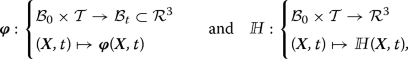(1)

where $${{\varvec{X}}}$$ denotes a material point at the microscopic reference configuration $${\mathcal {B}}_0$$, which is mapped to the current configuration $${\mathcal {B}}_t$$ by the deformation map $${\varvec{\varphi }}$$. The solvent-volume flux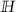describes the flow of the solvent volume relative to the solid skeleton. Such interpretation follows Biot’s approach to the modeling of porous media . For a detailed derivation of the equations used in the present contribution we refer to Coussy et al. .

Furthermore, we introduce the concentration of solvent volume describing the amount of solvent molecules in a referential infinitesimal volume element

\begin{aligned} s: {\left\{ \begin{array}{ll} {\mathcal {B}}_0\times {\mathcal {T}}\rightarrow {\mathcal {R}}^+ \\ ({{\varvec{X}}},t) \mapsto s({{\varvec{X}}},t) \end{array}\right. } \end{aligned}
(2)

with $$s = \nu c$$. Here, $$\nu$$ denotes the volume of a single solvent molecule and c is the number of these molecules. The solvent-volume concentration s can be related to the solvent flux by considering the conservation of solvent-volume concentration (Gurtin et al. ). It states that the change of solvent-volume concentration in a given arbitrary domain of a body is equal to the flux of solvent volume across the surface of that domain. Locally, this can be expressed as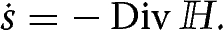(3)

Considering a first-order approach of homogenization, the independent fields can be decomposed into microscopic and macroscopic contributions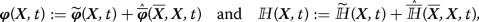(4)

where $${{\widetilde{{\varvec{\varphi }}}}}$$ anddenote microscopic fluctuations of the deformation map and the solvent-volume flux, respectively. We refer to Fig. 1 for a graphical illustration.

Further, considering a Taylor expansion of the macroscopic contributions up to the linear terms yieldsFootnote 3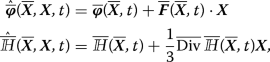(5)

where $${\overline{{{\varvec{F}}}}} = {\overline{{\text {Grad}}}}\,{\overline{{\varvec{\varphi }}}}$$ is the macroscopic deformation gradient and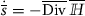is the rate of the macroscopic solvent-volume concentration. In what follows, we assume that the origin of the coordinate system is located at the geometric center of the RVE, i.e., $$\int _{{\mathcal {B}}_0} {{\varvec{X}}}\, \mathrm {d}V = {{\varvec{0}}}$$.

From (4) we obtain the deformation gradient $${{\varvec{F}}}$$ and the divergence of the solvent flux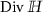as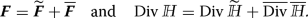(6)

The latter equation implies that the solvent-volume concentration can be decomposed into fluctuative microscopic and constant macroscopic parts as $$s = {\widetilde{s}} + {\overline{s}}$$. Integrating the equations in (6) over the domain of the RVE with $$|{\mathcal {B}}_0|=vol({\mathcal {B}}_0)$$ yields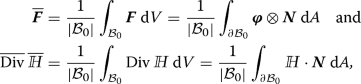(7)

where we have considered the following relations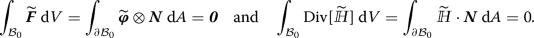(8)

The former equation is satisfied for continuous fluctuations of the deformation map along the whole microstructure, where continuity also relates to the transition across the (arbitrary) boundary of a periodic RVE. The restriction w.r.t. the continuity across an RVE’s boundary is usually expressed as $$\llbracket {{{\widetilde{{\varvec{\varphi }}}}}} \rrbracket = {{\varvec{0}}}$$ on $$\partial {\mathcal {B}}_0$$, where $$\llbracket {(\cdot )} \rrbracket =(\cdot )^+-(\cdot )^-$$ denotes the jump of a quantity $$(\cdot )$$ across the boundary of an RVE. Analogously equation (8)$$_2$$ is satisfied for continuous normal projections of the solvent-volume flux, i.e., anti-periodic normal projections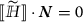on $$\partial {\mathcal {B}}_0$$. We refer to Fig. 2 for a graphical illustration.

### Constitutive functions at the microscopic level

As diffusion–deformation processes are dissipative in nature, they can be modeled by a set of two constitutive functions given by an energy-storage function $${{\hat{\psi }}}$$ and a dissipation-potential function $${{\hat{\phi }}}$$. The function $${{\hat{\psi }}}({{\varvec{F}}},s)$$ models the energy storage due to the deformation of the material and the influx of the solvent molecules. It is assumed to have the following additive form (Hong et al. [19, 20], Böger et al. )

\begin{aligned} {{\hat{\psi }}}({{\varvec{F}}},s) = {\hat{\psi }}_{mech}({{\varvec{F}}}) + {\hat{\psi }}_{chem}(s) + {\hat{\psi }}_{coup}(J,s) , \end{aligned}
(9)

where $${\hat{\psi }}_{mech}$$, $${\hat{\psi }}_{chem}$$ and $${\hat{\psi }}_{coup}$$ denote the mechanical, chemical and coupled chemo-mechanical contributions. For the modeling of hydrogels, a neo-Hookean material model can be considered for the mechanical energy $${\hat{\psi }}_{mech}$$. The chemical energy $${\hat{\psi }}_{chem}$$ is usually governed by a Flory–Rehner-type constitutive function (Flory and Rehner ). The term $${\hat{\psi }}_{coup}(J,s)$$ in the energy-storage function models the coupling between deformation and solvent volume and by relating the volume change of the material to the solvent-volume concentration s. In the variational minimization formulation to be developed below, the dissipation potential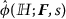is a convex function of the solvent flux.Footnote 4 The forms of these functions and their relevance for the specific problems at hand will be discussed in the following sections.

### Rate-type minimization principle of computational homogenization

The macroscopic potential densityof the homogenization problem at a given macroscopic constitutive state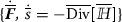can be described by the minimization principleFootnote 5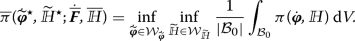(10)

This variational principle can be considered as a generalized Hill–Mandel condition and involves the minimization of the volume-averaged microscopic potential density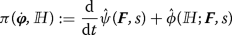(11)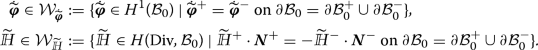(12)

### Incremental variational principle at the microscale

In the numerical setting, the above given rate-type variational principle (10) is solved incrementally at discrete times $$0<t_{n+1}\le T$$, where T denotes the ending time of a process. It is assumed that at the beginning of a time interval $$[t_n,\,t_{n+1}]$$ all variables are known. In the following, we consider the notation $$(\cdot )_n$$ for discrete variables at time $$t_n$$ and drop the subscript for the variables at time $$t_{n+1}$$, i.e., $$(\cdot ) := (\cdot )_{n+1}$$. Considering an implicit Euler time integration with a time step $$\tau = t_{n+1} - t_n$$, we replace (10) with an alternative incremental macroscopic potential density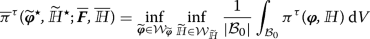(13)

in terms of the incremental microscopic potential density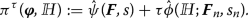(14)

Here, the solvent-volume concentration is determined via implicit Euler integration such that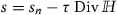. Analogously, we determine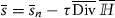at the macroscale. Note that in (14) the dissipation-potential function takes into account known state variables $$\{{{\varvec{F}}}_n,s_n\}$$ at time $$t_n$$ in order to preserve a variationally consistent structure of the problem (Miehe et al. , Böger et al. ). The admissible spaces in the incremental setting are defined as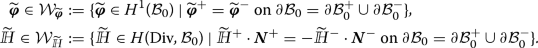(15)

### Euler–Lagrange equations and linearization of the variational formulation

The necessary condition of the incremental variational formulation (13) yields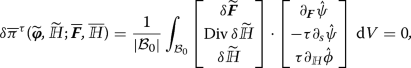(16)

where $$\delta {{{\widetilde{{\varvec{\varphi }}}}}} \in {\mathcal {W}}_{{{{\widetilde{{\varvec{\varphi }}}}}}}$$ and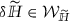. Using integral theorems as well as employing the periodicity conditions (15), we arrive at the incremental Euler–Lagrange equations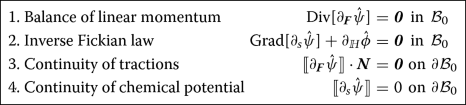(17)

In (17) we identify the constitutive equations for the microscopic dual fields, i.e., the first Piola–Kirchoff stress tensor $${{\varvec{P}}}:=\partial _{{\varvec{F}}}\hat{\psi }$$ and the chemical potential of the solvent-volume concentration $$\mu :=\partial _s{\hat{\psi }}$$. The equation (17)$$_{2}$$ describes an inverse form of Fick’s law. The driving force of the diffusion process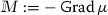is determined constitutively from the dissipation potential function via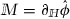.

Linearization of (16) leads to the following expression including the incremental microscopic moduli tensor(18)

In the following sections, we will take advantage of (16) and (18) when we consider the finite-element implementation of the homogenization problem.

### Finite-element implementation of the homogenization procedure

For the numerical solution of the microscopic diffusion–deformation problem, conforming quadrilateral $$\hbox {Q}_{1}\hbox {RT}_{0}$$ Raviart–Thomas-type finite elements are implemented . This choice is due to the required function space $$H({\text {Div}},{\mathcal {B}}_0)$$ of the solvent-volume flux. An alternative non-conforming discretization on the basis of standard $$\hbox {Q}_{1}\hbox {Q}_{1}$$ finite elements usually yields non-physical behavior. For recent developments of equivalent minimization principles incorporating a node-based finite-element discretization combined with reduced integration we refer to .

In the framework of the Raviart–Thomas-type finite-element discretization, the fluctuations of the deformation map $${{\widetilde{{\varvec{\varphi }}}}}$$ are approximated continuously using bilinear $$\hbox {Q}_{1}$$ shape functions, which are related to the nodal degrees of freedom $${{{\mathbf {\mathsf{{d}}}}}}{}_{{{\widetilde{{\varvec{\varphi }}}}}}^e$$ of a finite element. The fluctuations of the solvent-volume flux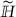are approximated considering linear $$\hbox {RT}_{0}$$ shape functions, which are related to the fluctuations of the flux degrees of freedom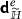across the edges of the quadrilateral element. We refer to Fig. 3a for a qualitative illustration.

Note that only the normal component of the solvent-volume flux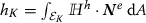at an edge of the element $$\mathcal {E}_K$$ is considered as degree of freedom. We refer to Raviart and Thomas , Brezzi and Fortin  for theoretical aspects and to Schwarz et al. , Anjam and Valdman , Böger et al. , Teichtmeister et al.  for discussions of the numerical implementation. The interpolation of the independent fields arises as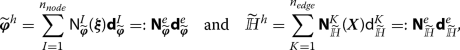(19)

where the scalar shape functions $$\mathsf{N}{}^I_{{{\widetilde{{\varvec{\varphi }}}}}}$$ are defined at the node I and the vectorial shape functionscorrespond to the edge K. While the former can be directly defined in a so-called parametric space $${\mathcal {A}}= [-1,\,1]\times [-1,\,1]$$, the latter have to be computed via a Piola transformation of the corresponding shape functionsin that parametric space (from here on the notation $$\widehat{(\cdot )}$$ indicates quantities $$(\cdot )$$ formulated in the parametric space). Similarly, $${{{\mathbf {\mathsf{{d}}}}}}{}^I_{{{\widetilde{{\varvec{\varphi }}}}}}$$ denotes the array of the displacement components at the nodeI and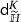corresponds to the normal component of the solvent-volume flux at the edge K. An illustration of the Raviart–Thomas shape functions in the parametric space $${\mathcal {A}}$$ is given in Fig. 4.

The $$\hbox {RT}_{0}$$ shape functions considered in the present contribution are given in the parametric space as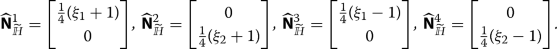(20)

The degrees of freedom need to be invariant under the change of coordinates from the parametric space to the physical space, i.e.,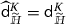. Thus, we apply a Piola transformation to map the vectorial shape functions and their derivatives from the parametric space $${\mathcal {A}}$$ to the reference element $${\mathcal {B}}{}_0^e$$. We refer to Brezzi and Fortin  for more details and to Fig. 3b for a graphical illustration. It follows

\begin{aligned} {[}\cdot ]({{\varvec{X}}}):={\mathcal {P}}\{[\cdot ]({\varvec{\xi }})\}=\frac{1}{\widehat{J}}\widehat{{{\varvec{J}}}}({\varvec{\xi }})[\cdot ](\xi ) \quad \text {with} \quad \widehat{{{\varvec{J}}}}:=\frac{\partial {{{\varvec{X}}}^h}}{\partial {\varvec{\xi }}} \quad { {\text{ and }}} \quad \widehat{J}:=\det \widehat{{{\varvec{J}}}} , \end{aligned}
(21)

where $${{{\varvec{X}}}^h}:{\mathcal {A}}\rightarrow {\mathcal {B}}{}_0^e$$ refers to a map from the parametric space to a reference finite element and is determined by $${{\varvec{X}}}^h({\varvec{\xi }}) = \sum _{I=1}^{n_{node}} \mathsf{N}^I_{{{\widetilde{{\varvec{\varphi }}}}}}({\varvec{\xi }}) {{\varvec{X}}}^I$$ in terms of the nodal coordinates $${{\varvec{X}}}^I$$ of the considered finite element.

Furthermore, it is important to point out that, in order to have a consistent finite-element implementation for the flux degrees of freedom, we need to make sure that the degrees of freedom on a common edge of two adjacent finite elements are identical. In order to achieve this, we assume a positive orientation for an edge in a finite element $${\mathcal {B}}^e$$ whenever the node numbers of the vertices of that edge increase in counterclockwise direction. In contrast, when the node number decreases, we augment the $$\hbox {RT}_0$$ shape function of that edge with a negative sign. As a consequence, an outflux at an edge of a particular finite element will always be associated with influx across the same edge of a neighboring finite element. We refer to Schwarz et al. , Anjam and Valdman , Böger et al. , Teichtmeister et al.  for more details on the numerical implementation.

Based on (19) we obtain the fluctuations of the deformation gradient and of the divergence of the flux fields as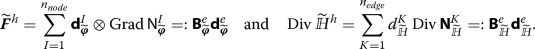(22)

Having the above discretization at hand, the necessary condition of the incremental minimization principle (16) in the finite-element setting can be written in form of the microscopic residuum vector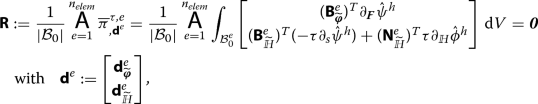(23)

where the first Piola–Kirchhoff stress tensor $${{\varvec{P}}}$$ is implemented as a vector, i.e., $${{{{\mathbf {\mathsf{{P}}}}}}}=[{P}_{11},\ {P}_{22},\ {P}_{12},\ {P}_{21}]^T$$ in two dimensions. The corresponding mechanical and coupled moduli tensors follow a similar structure. The tangent matrix of the linearized system of equations reads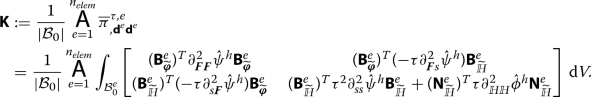(24)

A converged microscopic state is obtained by solving the system of equations (16) iteratively using, for example, a Newton–Raphson method

\begin{aligned} {{{{\mathbf {\mathsf{{d}}}}}}} \Leftarrow \begin{matrix} {{{{\mathbf {\mathsf{{d}}}}}}}&- {{{{\mathbf {\mathsf{{K}}}}}}}{}^{-1}&{{{\mathbf {\mathsf{{R}}}}}}\end{matrix} \ \quad \text {until} \quad ||{{{{\mathbf {\mathsf{{R}}}}}}}||\le tol_{micro} . \end{aligned}
(25)

### Effective macroscopic dual fields and moduli tensor

In the numerical setting, taking the variation of $${\overline{\pi }}{}^\tau$$ at the macroscopic material point yields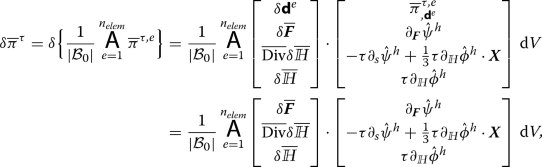(26)

where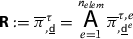vanishes at the solution point of the microscopic boundary-value problem. As a consequence, the macroscopic first Piola–Kirchhoff stress tensor $${\overline{{{\varvec{P}}}}}$$ and the negative gradient of the chemical potential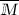(the latter being the driving force of the macroscopic solvent-volume flux) are determined as averages of the corresponding microscopic analogues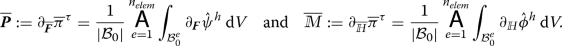(27)

However, the macroscopic chemical potential follows from a different averaging process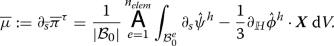(28)

We observe that $${\overline{\mu }}$$ does not only depend on the average of the microscopic chemical potential but also on its gradient and the spatial dimensions of the RVE. As a consequence, the macroscopic effective response depends on the size of the RVE, which will be discussed in detail in the next section.

Considering a continuous setting and exploiting tensorial manipulations in (27) and (28), we can express the effective dual fields in terms of the surface integrals over the boundary of the RVE as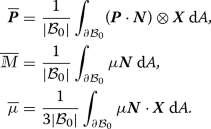(29)

Adopting the notation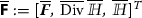, we can write the first-order terms in the linearization of (26) at the macroscale in a compact form as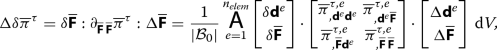(30)

where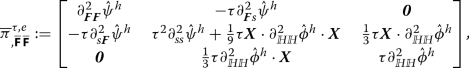(31)(32)

Combining (23), (26) and (30), we obtain an elimination equation at the macroscopic level for the microscopic degrees of freedom, that is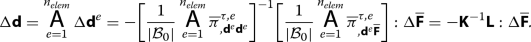(33)

Considering the results of the latter equation in (30), we obtain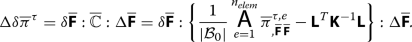(34)

wherein we have identified the macroscopic coupled moduli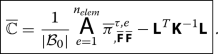(35)

Now having determined the macroscopic dual fields (27) and (28) as well as the macroscopic moduli (35), we could solve a macroscopic boundary value problem under prescribed boundary and initial conditions by using a conforming finite-element discretization analogous to the section “Finite-element implementation of the homogenization procedure”.

## Size effects in the homogenization of transient diffusion

In equations (28), (31) and (32), we observe terms that are scaled with the coordinates of the RVE. This gives rise to a size-dependent effective response at the macroscale. To have more insight into the problem, we simplify the above described formulation (10) to the diffusion in rigid solids by neglecting any elastic effects. Then, the problem at hand reads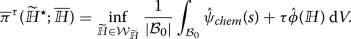(36)

To determine the macroscopic response of the material, we take the variation of (36) and arrive at the definition of the macroscopic chemical potential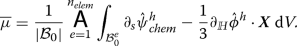(37)

Considering the dissipation-potential function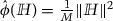for the diffusion process in rigid solids with mobility parameterFootnote 6M, we can reformulate the size-dependent term $${\overline{\mu }}_{\text {size}}$$ of the chemical potential $${\overline{\mu }}$$ in (37) by considering (4) and (5) as(38)

Furthermore, after some simple manipulations, we obtain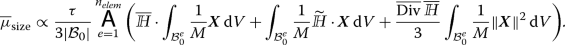(39)

We observe that the macroscopic effective response depends on the size of the RVE and the mobility parameter M. Note that the first and second term on the right-hand side of (39) vanish for specific realizations of RVEs.Footnote 7 In general, however, we expect variations among the effective responses depending on the particular selection of RVEs from periodic microstructures. Due to the size dependence, the effective response of a single unit cell $${\mathcal {D}}$$ differs from the effective response of an ensemble of identical unit cells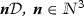. In the following, we provide some numerical examples that describe the mentioned size effect for RVEs with different sizes and material parameters.

## Representative numerical examples

In order to demonstrate the capabilities of the variational homogenization procedure discussed above, we present some numerical examples. The first numerical examples consider pure diffusion phenomena and investigate the influence of material parameters, geometry as well as the size of RVEs on the effective, macroscopic response of composite materials (see, the section “Investigation of the macroscopic properties of porous rigid solids”). In a second study, we extend the formulation by elastic effects of the solid matrix and couple it to the diffusion processes. In doing so, we will investigate swelling-induced pattern transformations of periodic hydrogels (see, the section “Coupled diffusion—deformation processes in periodic hydrogels”).

In what follows, we assume that the relaxation time of the material is much longer than the solvent’s diffusion time. We thus neglect any viscoelastic effects of the material and consider standard Fickian-type diffusion [8, 19, 20]. For an implementation that takes into account the viscoelasticity of the material we refer to Govindjee and Simo .

### Investigation of the macroscopic properties of porous rigid solids

In this subsection, we analyze the influence of material parameters and the size of RVEs on the macroscopic response of two-phase materials. We will find that the macroscopic mobility parameter lies within the classical Voigt and Reuss bounds for vanishing RVE size.

#### Microscopic constitutive functions

In the section “Constitutive functions at the microscopic level”, we have discussed the basic forms of the energy-storage and dissipation-potential function. In case of a pure diffusion processes (for $${\varvec{\varphi }}= \mathrm {const}.$$ with $${{\varvec{F}}}= {{\varvec{1}}}$$), the energy-storage function reduces to $${\hat{\psi }}(s) = {\hat{\psi }}_{chem}(s)$$. As our main focus is to investigate fundamental effects, we select the simple quadratic function (Kaessmair and Steinmann )

\begin{aligned} {\hat{\psi }}_{chem}(s) = \frac{A}{2}\left( s-\frac{1}{2}\right) ^2 , \end{aligned}
(40)

where A is a chemical material parameter. As dissipation-potential function we again considerwith the mobility parameter M.

#### Description of the problem

In the following, we consider periodic microstructures with circular inclusions. For such microstructures it is straightforward to indentify a periodic unit cell (see Fig. 5a). In a typical homogenization framework, the effective response of the material could be computed uniquely by considering an RVE that resembles exactly that unit cell. However, as we could see in the section “Size effects in the homogenization of transient diffusion”, the homogenization of transient diffusion processes comes along with well-known size effects in the sense that the physical size of the considered RVE influences its effective response.

In order to analyze this size effect, we will alter the size of associated RVEs in the following way. On the one hand, we will change the size by adjusting the lateral length of the underlying unit cell. On the other hand, we will change the size by composing an RVE of a certain number of unit cells. In both cases the RVEs will be generated from a primitive square-shaped unit cell having the lateral length of 1 mm. We adopt the following name convention: ’nRVEmm’ designates a unique realization of an RVE, where n indicates the scaling of the dimensions of the primitive unit cell and mm indicates a pattern of $$m \times m$$ primitive unit cells. For example, 2RVE11 and RVE22 both represent an RVE with side length of 2 mm, but while the former is realized by scaling the primitive unit cell by a factor of 2, the latter is made up of $$2 \times 2$$ primitive unit cells. Please refer to Fig. 8 for a corresponding visualization. In all following simulations, we consider a volume fraction of the inclusions of $$f_0=\pi /16$$.

In a purely diffusive process, the RVEs can be driven by the macroscopic solvent-volume flux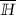and its divergence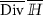. As the size effect is due to the linear terms appearing in the solvent flux vector in (5)$$_{2}$$ (see also (39)), we take into account the following macroscopic loading conditions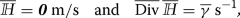(41)

where $${\overline{\gamma }}$$ is a loading parameter. In the current case, we assume that the divergence of the macroscopic solvent-volume flux increases linearly with time, i.e, $${\overline{\gamma }}\propto t$$, see Fig. 5b. All simulations are carried with the time incrementation $$\tau =0.01$$ s. The chemical parameter of the matrix and the inclusion is selected as $$A_{mat} = A_{incl} = 10\,\hbox {N/mm}^{2}$$. Furthermore, at the beginning of the simulation, the solvent-volume concentration at micro- and macroscale is assumed as $$s(t = t_0) = {\overline{s}}(t = t_0) = 0$$. Dirichlet boundary conditions for the normal projections of the fluctuations of the solvent-volume flux are considered, i.e., we setFootnote 8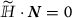on $$\partial {\mathcal {B}}_0$$.

#### Influence of mobility parameters on the effective macroscopic response

We now investigate the influence of mobility parameters of the individual constituents on the macroscopic response of the two-phase material.

In a first step, we consider different kinds of square-shaped RVEs with constant mobility parameter $$M_{mat}=0.1\,\hbox {mm}^{4}$$/(Ns) of the matrix and altering mobility parameters of the inclusions.

In Fig. 6a we observe discrepancies in the results depending on the realization of the respective RVE. An increasing size of the RVE leads to a higher magnitude of the effective chemical potential. This effect is due to the last term in (39), which arises as the result of the transient nature of the problem. We refer to Larsson et al.  for a similar analysis. As expected from (39), the discrepancies of the effective responses of different RVEs decrease when we increase the mobility parameter of the inclusions. However, since we keep the mobility parameter of the matrix unchanged at a comparably low level ($$M_{mat}=0.1\,\hbox {mm}^{4}$$/(Ns)) the size effect is still pronounced. Further comparing the responses of 2RVE11 and RVE22, we observe that when the mobility parameter of the matrix and the inclusion have a similar magnitude, the corresponding effective responses nearly coincide (Fig. 6b). In fact, if we would assume $$M_{mat} \equiv M_{incl}$$, the responses would be identical since then the RVEs are homogeneous.

In Fig. 7, we show the influence of the mobility parameter of the matrix material on the effective response for fixed mobility parameter of the inclusions $$M_{incl}=10^{-4}\,\hbox {mm}^{4}$$/(Ns). We observe decreasing discrepancies in the effective responses with increasing mobility parameter of the matrix. This effect can be attributed to the higher volume fraction of the matrix.

Contour plots of the microscopic chemical potential $$\mu$$ for different realizations of RVEs are shown in Fig. 8. Remarkable differences in the microscopic response of the RVEs are evident. This confirms that the underlying periodicity cannot be used to reduce computations over an enlarged RVE to computations over a unit-cell RVE.

Above we have demonstrated that the effective chemical potential strongly depends on the mobility parameters of the individual phases as well as on the size and realization of the RVE. We now take a close look at the evolution of the chemical potential for various RVEs over time (Fig. 9). In analogy to the previous examples, we consider square RVEs that are either made up of a certain number of equal-sized unit cells or made up of a scaled-up unit cell. The macroscopic loading is again formulated in terms of the loading parameter $${\overline{\gamma }}$$, which will first be linearly decreased to a minimum value and then increased to zero. After that, it will be kept fixed. Please refer to Fig. 5c for a graphical illustration, in which the negative sign of the loading parameter is related with an influx of the solvent. For time incrementation we consider the time step $$\tau =0.1\,\text {s}$$.

In Fig. 9a we observe that the different RVEs show the expected differences in their initial effective response. However, after a certain period of time the responses of all RVEs converge to the same stationary solution, where low mobility parameters of the inclusions lead to longer relaxation times (compare Fig. 9a–c). We further observe that an increase of the mobility parameter of the inclusions has negligible influence on the behavior under loading, but strongly influences the unloading phase (compare Fig. 9a,d with Fig. 9b,e respectively). In contrast to that, changing the mobility parameter of the matrix strongly influences the loading phase, but shows qualitatively similar trends in the unloading regime (compare Fig. 9a,d with Fig. 9c,f respectively). As a final observation we note that the stationary solutions of different RVEs converge to the same values, even when the material parameters of the individual phases are different. (compare Fig. 9b with Fig. 9e). This behavior could be expected from the size-dependent contribution of the effective chemical potential given in (39). There, the first term has been assumed zero per definition and the last term of the right-hand side is zero after full unloading. As a consequence, the second term fades out over time due to internal redistributions of the solvent such that finally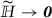and thus $${\overline{\mu }}{}_{\text {size}}\rightarrow 0$$. In the stationary state, the size-independent term of the macroscopic chemical potential (37) is identical for all considered RVEs since we assume the same chemical parameters for both phases in the microscopic chemical energy (40).

#### Influence of the size of the RVE on the macroscopic moduli

Next, we study the effect of the size of the RVE on the resulting macroscopic moduli. The influence of the size of the RVE for $$M_{mat}=10^{-1}\,\hbox {mm}^{4}$$/(Ns) and $$M_{incl}=10^{-4}\,\hbox {mm}^{4}$$/(Ns) on the effective response is shown in Fig. 10

We observe that as the size of the RVE increases the macroscopic chemical parameter $$\partial ^2_{{\overline{s}}\,{\overline{s}}}{\overline{\pi }}$$ as well as the macroscopic mobility parameter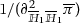(see (35)), increase monotonically although the microscopic material parameters are not changed. However, for $$l_{micro}\rightarrow 0$$, the macroscopic moduli saturate. As becomes visible in Fig. 10a, the macroscopic chemical parameter approaches the average value of the microscopic chemical parameters, i.e., $$\langle \partial ^2_{ss}\psi \rangle :=\frac{1}{ {\mathcal {B}}_0|} \int _{{\mathcal {B}}_0} \partial ^2_{ss} \psi \, \mathrm {d}V = 10~\hbox {N/mm}^{2}$$. Analogously, in Fig. 10b, the macroscopic mobility parameter for $$l_{micro}\rightarrow 0$$ is bounded by the classical Voigt and Reuss bounds (Zohdi and Wriggers )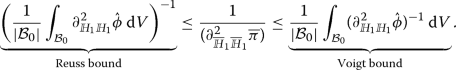(42)

To further study the influence of the size of the RVE depending on the mobility parameter of the microstructure, we provide three-dimensional plots for the different mobility parameters of the matrix $$M_{mat}=\{0.001,\,0.1,\,1.0\}~\hbox {mm}^{4}$$/(Ns) in Fig. 11.

We plot the macroscopic chemical parameter and the macroscopic mobility parameter against RVE size ($$l_{micro}\in [0.002,2.0]$$ m) and mobility parameter of inclusions ($$M_{incl}\in [0.001,10]\,\hbox {mm}^{4}$$/(Ns)) in Fig. 11a–c and d–f, respectively.

In Fig. 11a–c, we observe that the macroscopic chemical parameter is highly dependent on the RVE size $$l_{micro}$$ as well as the mobility parameter of the matrix material $$M_{mat}$$. As $$M_{mat}$$ increases the macroscopic chemical parameter decreases. However, the influence of the mobility parameter of the inclusions is lower than the one of the matrix material. In Fig. 11c, we see that as $$M_{incl} \rightarrow 0$$ the effective chemical parameter increases in the given range. In all instances of $$M_{mat}$$, $$M_{incl}$$ and $$l_{micro}$$, the macroscopic chemical parameter approaches $$10~\hbox {N/mm}^{2}$$ when the RVE size approaches zero.

In Fig. 11d–f, we observe that the macroscopic mobility parameter is highly dependent on the size of the RVE and the mobility parameter of the matrix. We also see that for lower values of matrix mobility parameter, the influence of the mobility parameter of the inclusions becomes more pronounced. Additionally, the RVE size becomes more relevant for increasing magnitudes of $$M_{mat}$$.

Finally, in Fig. 11g–i we illustrate regions of macroscopic mobility parameter that are bounded from above by the classical Voigt bound (42). It is evident that low magnitudes of matrix mobility parameter lead to larger regions bounded by the Voigt bound. The size of the RVE has a similar influence on this region.

### Coupled diffusion–deformation processes in periodic hydrogels

We now present a numerical example that demonstrates the coupled chemo-mechanical response of a two-phase periodic hydrogel microstructure. To be specific, we analyze a pattern transformation due to diffusion-induced swelling phenomena (we refer to Zhu et al.  for experimental evidence). In order to model such phenomenon, we need to consider appropriate constitutive equations that take into account the coupled response of hydrogels. The subsequent sections are thus first devoted to the discussion of the used microscopic constitutive functions. Thereafter we analyze the behavior of a periodic hydrogel in consideration of typical geometrical and physical properties under given macroscopic loading conditions.

#### Microscopic constitutive functions

We assume that the mechanical contribution of the energy-storage function in (9) can be described by a neo-Hookean function. The chemical energy function is considered to be of the Flory–Rehner-type. These functions are given as

\begin{aligned} {\hat{\psi }}_{mech}({{\varvec{F}}})=\frac{\gamma }{2} ( {{\varvec{F}}}:{{\varvec{F}}}- 3 -2\ln J ) \quad {\text{ and }} \quad {\hat{\psi }}_{chem}(s) = \alpha \left[ s\ln \left( \frac{s}{1+s} \right) +\frac{\chi s}{1+s} \right] , \end{aligned}
(43)

where $$\alpha$$ is a mixing modulus and $$\chi$$ is a dimensionless Flory–Huggins interaction parameter. The coupling of the mechanical and the chemical response of the material is modeled with the function

\begin{aligned} {\hat{\psi }}_{coup}(J,s) = \frac{\epsilon }{2} ( J - 1 - s)^2 , \end{aligned}
(44)

where $$\epsilon$$ is a penalty parameter enforcing the volumetric constraint $$J=1+s$$, see Böger et al. . The dissipation-potential function is a convex function of the solvent flux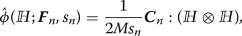(45)

where $${{\varvec{C}}}$$ is the right Cauchy–Green tensor and M is a mobility parameter (Böger et al. ).

We note that the swelling-volume concentration $$s\ge 0$$ is a non-negative quantity and $$s = 0$$ corresponds to a dry hydrogel polymer network. However, due to the present singularity of (43)$$_{2}$$ at the dry state we employ a stress-free pre-swollen state as the reference configuration for the Flory–Rehner energy function. We refer to Hong et al. [19, 20], Böger et al.  for detailed discussions. Consequently, we write the final forms of the energy-storage function

\begin{aligned} {\hat{\psi }} = \frac{\gamma }{2J_0} [ J_0^{2/3} {{\varvec{F}}}: {{\varvec{F}}}- 3 - 2 \ln (JJ_0) ] + \frac{\alpha }{J_0} \left[ s \ln \left( \frac{s}{1+s} \right) + \frac{\chi s}{1+s} \right] + \frac{\epsilon }{2 J_0} ( J J_0 - 1 - s )^2 , \end{aligned}
(46)

and the dissipation-potential function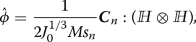(47)

where $$J_0$$ is a Jacobian that characterizes the volume change due to uniform pre-swelling. The initial solvent-volume concentration is determined from the assumption of a stress-free reference configuration as

\begin{aligned} s_0 = \frac{\gamma }{\epsilon }\big (J_0^{-1/3}-\frac{1}{J_0} \big ) + J_0 -1 . \end{aligned}
(48)

The macroscopic solvent concentration $${\overline{s}}_0$$ at the pre-swollen state is defined as the average of the microscopic solvent-volume concentration $$s_0$$.

#### Description of the problem

To analyze the effective chemo-mechanical behavior of a hydrogel we consider a two-phase square-shaped RVE with size $$l_{micro} = 2~\mathrm {mm}$$ build out of four periodic unit cells (the unit cell is depicted in Fig. 5). The volume fraction of the inclusions is $$\pi /16$$. The inclusions are softer than the matrix and have a higher mobility parameter. The material parameters are listed in Table 1.

The RVE is loaded with the macroscopic fields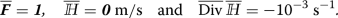(49)

in consideration of periodic boundary conditions for the fluctuations of the deformation map $${\widetilde{{\varvec{\varphi }}}}$$ and homogeneous Dirichlet boundary conditions for the fluctuations of the outward normal flux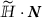. The computations are performed with a time incrementation of $$\tau = 0.1$$ s. To ensure that the problem is well-defined, we fix the fluctuations of the deformation map at the corner nodes of the RVE.

#### Swelling-induced pattern transformation of a periodic hydrogel

As a numerical example, we analyze a swelling-induced pattern transformation of a two-phase periodic hydrogel. The periodic RVE is discretized by using 16, 000 $$\hbox {Q}_{2}\hbox {RT}_{0}$$ finite elements. In Fig. 12, we illustrate contour plots of the $$P_{11}$$-component of the first Piola–Kirchhoff stress at three different time instances.

We observe that initially the soft inclusions having a volume fraction of $$\pi /16$$ shrink uniformly until a critical loading point is reached. At critical loading, the RVE undergoes a pattern transformation in the form of a buckling mode. A similar behavior has been observed in experiments by Zhu et al. . When the microstructure buckles the shape of the inclusions becomes non-circular, a state which is typically referred to as the diamond-plate pattern . Such an instability mode has also been observed by Bertoldi et al.  for periodic elastic structures with voids or soft inclusions under purely compressive loading. To trigger the buckling mode shown in Fig. 12, we have slightly perturbed the finite-element mesh in the given directions.

## Summary

We provided a computational homogenization framework for diffusion–deformation processes within a variationally consistent minimization-based setting that takes into account the deformation map and solvent-volume flux as independent field variables. We have discussed the theoretical aspects as well as the finite-element implementation of the formulation. The latter was realized by means of a conforming Raviart–Thomas-type discretization. By doing so, we were able to compute the macroscopic dual fields and incremental moduli in the numerical setting. Consistent with previous works from the literature, we found that the formulation yields a size-dependent macroscopic response. We confirmed this effect in a number of numerical examples by consideration of different RVEs with different mobility parameters and sizes. We further confirmed that the macroscopic mobility properties are bounded by the classical Reuss and Voigt bounds when the RVE size approaches zero. We also presented a numerical example showing a swelling-induced buckling mode of a soft periodic hydrogel.

## Data availibility

Data will be made available on request.

1. A higher-order approach has been proposed by Kouznetsova et al. .

2. We refer to Pham et al.  for the homogenization of microscopically transient problems in the framework of dynamic metamaterials.

3. We note that in (5)$$_{2}$$, we have assumed macroscopic isotropic swelling. Consequently, the linear expansion of the solvent-volume flux is associated with the divergence and not the gradient of. From the following equations, it will become clear that the considered form of the microscopic flux preserves the well-known structure of the diffusion–deformation problem at the micro- and macroscale.

4. Convexity of the dissipation potential function together with vanishing first derivatives at the origin guarantee a priori fulfillment of the second law of thermodynamics.

5. The index $$(\cdot ){}^\star$$ denotes a converged solution. To arrive at a compact notation, we will drop this index in the following whenever there is no danger of confusion.

6. The mobility parameter M can be computed via the Stokes–Einstein relation

\begin{aligned} M = \frac{\nu D}{k_B T} = \frac{\nu }{6\pi R\eta } , \end{aligned}

where $$\nu$$ is the volume of a single solvent molecule, D is the diffusivity, $$k_B$$ is the Boltzmann constant, T is the absolute temperature, R is the radius of the solvent molecules and $$\eta$$ is the viscosity of the solvent [10, 20].

7. One such realization is given by a symmetric RVE that is parameterized with coordinate axes that originate from the spatial center of the RVE.

8. We note that in the Raviart–Thomas finite-element implementation anti-periodic boundary conditions need to be considered for the flux degrees of freedom across the boundary of the RVE in order to guarantee a continuous solvent normal flux throughout the microstructure. This can be achieved, for example, by augmenting the shape functions corresponding to the edge-based degrees of freedom at a boundary point $${{\varvec{X}}}^+$$ on $$\partial {\mathcal {B}}^+$$ with an opposite sign compared with the shape functions at $${{\varvec{X}}}^-$$ on $$\partial {\mathcal {B}}^-$$.

## References

1. Anjam I, Valdman J. Fast matlab assembly of fem matrices in 2d and 3d: edge elements. Appl Math Comput. 2015;267:252–63.

2. Bertoldi K, Boyce M, Deschanel S, Prange S, Mullin T. Mechanics of deformation-triggered pattern transformations and superelastic behavior in periodic elastomeric structures. J Mech Phys Solids. 2008;56:2642–68.

3. Biot MA. General theory of three-dimensional consolidation. J Appl Phys. 1941;12(2):155–64.

4. Böger L, Nateghi A, Miehe C. Minimization-and saddle-point-based modeling of diffusion-deformation-processes in hydrogels. PAMM. 2016;16(1):307–8.

5. Brezzi F, Fortin M. Mixed and hybrid finite element methods. Berlin: Springer; 1991.

6. Chatzigeorgiou G, Charalambakis N, Chemisky Y, Meraghni F. Periodic homogenization for fully coupled thermomechanical modeling of dissipative generalized standard materials. Int J Plast. 2016;81:18–39.

7. Chen J, Park K. Synthesis and characterization of superporous hydrogel composites. J Control Release. 2000;65(1–2):73–82.

8. Chester SA, Anand L. A coupled theory of fluid permeation and large deformations for elastomeric materials. J Mech Phys Solids. 2010;58(11):1879–906.

9. Coussy O, Dormieux L, Detournay E. From mixture theory to Biot’s approach for porous media. Int J Solids Struct. 1998;35(34–35):4619–35.

10. Edward JT. Molecular volumes and the Stokes–Einstein equation. J Chem Educ. 1970;47(4):261.

11. Ehlers W, Wagner A. Multi-component modelling of human brain tissue: a contribution to the constitutive and computational description of deformation, flow and diffusion processes with application to the invasive drug-delivery problem. Comput Methods Biomech Biomed Eng. 2015;18(8):861–79.

12. Feyel F, Chaboche J-L. FE$$^2$$ multiscale approach for modelling the elastoviscoplastic behaviour of long fibre SiC/Ti composite materials. Comput Methods Appl Mech Eng. 2000;183:309–30.

13. Flory PJ, Rehner JJ. Statistical mechanics of cross-linked polymer networks ii. swelling. J Chem Phys. 1943;11(11):521–6.

14. Geymonat G, Müller S, Triantafyllidis N. Homogenization of nonlinearly elastic materials, microscopic bifurcation and macroscopic loss of rank-one convexity. Arch Ratl Mech Analy. 1993;122:231–90.

15. Govindjee S, Simo JC. Coupled stress-diffusion: case II. J Mech Phys Solids. 1993;41(5):863–87.

16. Gurtin ME, Fried E, Anand L. The mechanics and thermodynamics of continua. Cambridge: Cambridge University Press; 2010.

17. Hashin Z, Shtrikman S. A variational approach to the theory of the elastic behaviour of multiphase materials. J Mech Phys Solids. 1963;11:127–40.

18. Hill R. The elastic behaviour of a crystalline aggregate. Proc Phys Soc A. 1952;65:349–54.

19. Hong W, Liu Z, Suo Z. Inhomogeneous swelling of a gel in equilibrium with a solvent and mechanical load. Int J Solids Struct. 2009;46(17):3282–9.

20. Hong W, Zhao X, Zhou J, Suo Z. A theory of coupled diffusion and large deformation in polymeric gels. J Mech Phys Solids. 2008;56(5):1779–93.

21. Kaessmair S, Steinmann P. Computational first-order homogenization in chemo-mechanics. Arch Appl Mech. 2018;88(1–2):271–86.

22. Kouznetsova VG, Geers MGD, Brekelmans WAM. Multi-scale second-order computational homogenization of multi-phase materials: a nested finite element solution strategy. Comput Methods Appl Mech Eng. 2004;193:5525–50.

23. Krischok A, Linder C. A generalized inf-sup test for multi-field mixed-variational methods. Comput Methods Appl Mech Eng. 2019;357:112497.

24. Larsson F, Runesson K, Su F. Variationally consistent computational homogenization of transient heat flow. Int J Num Methods Eng. 2010;81(13):1659–86.

25. Miehe C, Mauthe S, Teichtmeister S. Minimization principles for the coupled problem of darcy-biot-type fluid transport in porous media linked to phase field modeling of fracture. J Mech Phys Solids. 2015;82:186–217.

26. Miehe C, Schotte J, Schröder J. Computational micro-macro transitions and overall moduli in the analysis of polycrystals at large strains. Comput Mater Sci. 1999;16:372–82.

27. Nemat-Nasser S, Hori M. Micromechanics: overall properties of heterogeneous materials, Vol. 36 of North-Holland series in Applied Mathematics and Mechanics. 2nd ed. New Jersey: Elsevier Science Publisher B. V; 1999.

28. Nilenius F, Larsson F, Lundgren K, Runesson K. Computational homogenization of diffusion in three-phase mesoscale concrete. Comput Mech. 2014;54(2):461–72.

29. Özdemir I, Brekelmans W, Geers M. Computational homogenization for heat conduction in heterogeneous solids. Int J Num Methods Eng. 2008;73(2):185–204.

30. Pham K, Kouznetsova VG, Geers MG. Transient computational homogenization for heterogeneous materials under dynamic excitation. J Mech Phys Solids. 2013;61(11):2125–46.

31. Polukhov E, Vallicotti D, Keip M-A. Computational stability analysis of periodic electroactive polymer composites across scales. Comput Methods Appl Mech Eng. 2018;337:165–97.

32. Raviart PA, Thomas JM. Primal hybrid finite element methods for 2nd order elliptic equations. Math Comput. 1977;31(138):391–413.

33. Reuss A. Berechnung der Fließgrenze von Mischkristallen aufgrund der Plastizitätsbedingung für Einkristalle. Zeitschrift für angewandte Mathematik und Mechanik. 1929;9:49–58.

34. Schwarz A, Schröder J, Starke G. A modified least-squares mixed finite element with improved momentum balance. Int J Num Methods Eng. 2010;81(3):286–306.

35. Teichtmeister S, Mauthe S, Miehe C. Aspects of finite element formulations for the coupled problem of poroelasticity based on a canonical minimization principle. Computational Mechanics. 2019;1–32.

36. Temizer I. On the asymptotic expansion treatment of two-scale finite thermoelasticity. Int J Eng Sci. 2012;53:74–84.

37. Temizer I, Wriggers P. Homogenization in finite thermoelasticity. J Mech Phys Solids. 2011;59(2):344–72.

38. Truskey GA, Yuan F, Katz DF. Transport phenomena in biological systems 2004

39. Voigt W. Über die Beziehung zwischen den beiden Elastizitätskonstanten isotroper Körper. Annalen der Physik. 1887;38:573–87.

40. Wang J, Yang J, Xie J, Xu N. A novel conductive polymer-sulfur composite cathode material for rechargeable lithium batteries. Adv Mater. 2002;14(13–14):963–5.

41. Wang L, Ueda T. Mesoscale modeling of water penetration into concrete by capillary absorption. Ocean Eng. 2011;38(4):519–28.

42. Zhu X, Wu G, Dong R, Chen C-M, Yang S. Capillarity induced instability in responsive hydrogel membranes with periodic hole array. Soft Matter. 2012;8(31):8088–93.

43. Zohdi T, Wriggers P. On the effects of microstress on macroscopic diffusion processes. Acta Mechanica. 1999;136(1–2):91–107.

Not applicable.

## Funding

The financial support of the German Research Foundation (DFG) in the framework of the Collaborative Research Center SFB 1313 (327154368) and within the Cluster of Excellence EXC 2075 (390740016) at the University of Stuttgart is gratefully acknowledged.

## Author information

Authors

### Contributions

EP and M-AK developed the research idea and designed the study. EP performed the numerical implementation and post-processed the simulation data. EP and M-AK interpreted the results. Both authors wrote the manuscript and gave their final approval for publication. Both authors read and approved the final manuscript.

### Corresponding author

Correspondence to Marc-André Keip.

## Ethics declarations

### Competing interests

The authors have no competing interests.